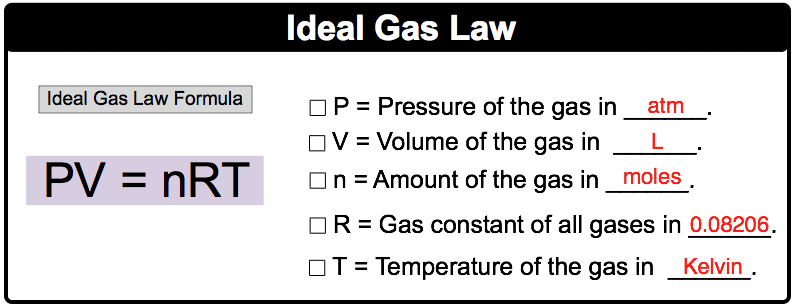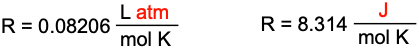Clutch Prep is now a part of Pearson
Ch.8 Gases, Liquids and SolidsWorksheetSee all chapters

# The Ideal Gas Law

See all sections
Sections
Pressure Units
Kinetic Molecular Theory
The Ideal Gas Law
The Ideal Gas Law Derivations
The Ideal Gas Law Applications
Chemistry Gas Laws
Chemistry Gas Laws: Combined Gas Law
Standard Temperature and Pressure
Dalton's Law: Partial Pressure (Simplified)
Gas Stoichiometry
Intermolecular Forces (Simplified)
Intermolecular Forces and Physical Properties
Atomic, Ionic and Molecular Solids
Heating and Cooling Curves

The Ideal Gas Law formula relates the behavior of gases under varying conditions of pressure, volume, moles and temperature.

###### The Ideal Gas Law

Concept #1: The Ideal Gas LawExample #1: A 500 mL container at a pressure of 600 mmHg possesses 29.3 g nitrogen gas at 50 ºC. Determine the correct units needed for the Ideal Gas Law.

Concept #2: R ConstantExample #2: How many moles of NH3 are contained in a 25.0 L tank at 190 ºC and 5.20 atm?

Practice: How many grams of carbon dioxide, CO2, are present in a 0.150 L flask recorded at 525 mmHg and 32 ºC?

a) 1.77 g

b) 0.93 g

c) 0.66 g

d) 0.18 g

e) 0.052 g

Practice: How many liters of HNO3 gas, measured at 28.0 ºC and 780 torr, are required to prepare 2.30 L of 4.15 M solution of nitric acid?

Practice: When 0.670 g argon is added to a 500 cm3 container with a sample of oxygen gas, the total pressure of the gases is found to be 1.52 atm at a temperature of 340 K. What is the mass of the oxygen gas in the bulb?